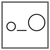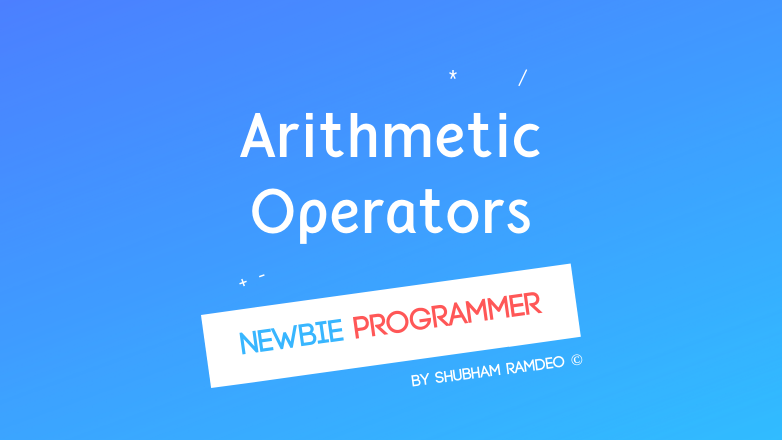Fuddlepixel coz tech is fun();

# Arithmetic Operators

Let us discuss the arithmetic operators through which we can perform mathematical operations in the C programming language.

By Shubham Ramdeo
18 August 2015 · 5 mins read
# `c`  `newbie`How can a computer performs mathematics ? It is the arithmetic operators !

Welcome to the Newbie Programming Series. This is the first part of our new topic, Operators. Yet I am doing it in a separate fashion because we have got too many things to be remembered.  So In this part, we will discuss the arithmetic operators and their operations. You are already used to with them. Addition, subtraction etc. But lets discuss in a little detail. If you are new to this series, please go the Index and read out all the previous parts.

## The Arithmetic Operators

Alright, Now the Arithmetic Operators. Arithmetic is simple maths, its simple calculation that we do with two numbers. Below is the list of the operators available in the C programming language that can be used for arithmetic.

# Let A = 10 and B = 5

To add, use “+

eg. A + Bgives 10

#### Subtraction

To subtract, use “ - “

eg. A - B gives 5

#### Multiplication

To multiply, use “ * “

eg. A * B gives 50

TIP: Its good if you keep the mark separate. Like if you are doing A * B then its correct. BUT doing A* B or A *B might create some troubles. Avoid using such way.

#### Division

To divide, use “ / “

Here division by zero is not allowed and will cause errors.

eg. A / B gives 2

TIP: Sometimes we put a double / instead of one. So instead of writing for example : A + C / B + ( 6 * B + 9 * B ) , we write A + C // B ( 6 * B + 9 * B ) And we know that // is used for one line comments. So In the above incorrect statement, A + C // B ( 6 * B + 9 * B ) , It will mean only A + C as all the rest has been skipped due to // (as a comment)

#### Modulus

To find the reminder when B divides A, use “ % “

eg. A % B gives 0

#### Increments

To increase the value by 1, use “ ++ “

eg. A++ gives 11

It actually means A = A + 1

#### Decrements

To decrease the value by 1, use “ – “

eg. B– gives 5

It actually means B = B - 1

The above increments and decrements operators are very and mostly useful during loops.

### THE INCREMENT DECREMENT AFTER or BEFORE VARIABLE DOUBT SOLVED

#### There is a little doubt which I have seen everywhere. What is the difference between ++x and x++ ? Lets Discuss.

Both mean the same if used stand alone. Both will increase the value of the variable by 1. But the use of this difference lies in the way the program flows.

For example:

The first one is with a++

``````#include <stdio.h>
main()
{
int a, b;
a = 3;
b = a++; //note
printf("a is : %d \n", a);
printf("b is : %d \n", b);

return(0); //to exit the program
}
``````

OUTPUT:

a is : 4

b is : 3

Now Do the other one. With ++a

``````#include <stdio.h>
main()
{
int a, b;
a = 3;
b = ++a; //note
printf("a is : %d \n", a);
printf("b is : %d \n", b);

return(0); //to exit the program
}
``````

OUTPUT:

a is 4

b is 4

Do you got the difference ?

Actually a++ means “remember a then increase it”

But, ++a means “increase it then remember a”

So in the above programs, when we used b = a++ It means b is equal to : remember a then increase it by 1. So b get the original value of a, 3 and THEN a becomes 4. While when we used b = ++a It means b is equal to : increase a then remember it. So first a gets increased to 4. And this new value is used in b. Read the program again. The way the program is flowing, you will now easily understand the difference.

Just remember :

a++ means “remember a then increase it”

++a means “increase it then remember a”

SAME IS POSSIBLE WITH decrements –a or a–

BUT things like ++a++ or –a– ARE NOT ALLOWED !!!

### Tricks to do arithmetic with “equal to” Shortcut

Sometimes we just want to change the value of original variable. Use the following table to use the shortcuts:

FOR     x = x + n     USE    x+=n

FOR     x = x - n      USE    x-=n

FOR     x = x * n     USE    x*=n

FOR     x = x / n      USE    x/=n

FOR     x = x % n    USE    x%=n

This could save a lot of time.

NOTE:  When we use the = operator, the = character comes last.

So let if A is 20, if you do A-=5 ,the value of A will decrease by 5 and A becomes 15

BUT if you did A=-5 , A will become -5 !!!

Well I don’t find any program that is useful to make just now, as we have already made a calculator which can do simple calculation. But it doesn’t contain the reminder operator so I added and made a new one down below:

``````#include  <stdio.h>
main()
{
float a, b;

printf("Please enter two numbers with a space between them like 4.2 6.5 \n");
printf("BUT don't put the second one zero as division by zero is not possible.\n");
scanf("%f %f", &a, &b);

printf("Sum is %f \n". a + b);
printf("Subtraction is %f \n", a - b);
printf("Multiplication is %f \n", a * b);
printf("Division is %f \n", a / b );
printf("Reminder is %f \n", a % b );
getchar();
}
``````

Anyways, with time, coding will sometimes make you scratch your head a bit but don’t worry. Contact me on fb twitter, I will surely solve your troubles. We are gonna discuss Relational Operators from the next post. Stay connected.

##### Update me weekly ✉

Subscribe below and we’ll send you a weekly email summary of all new Code tutorials. Never miss out on learning about the next big thing.

#### Shubham RamdeoShubham Ramdeo is the founder of Fuddlepixel. He is a geek, a writer, involved with many technical and humanity projects.

Meet me!

#### Recent#### Related

`c`

`newbie`

Top